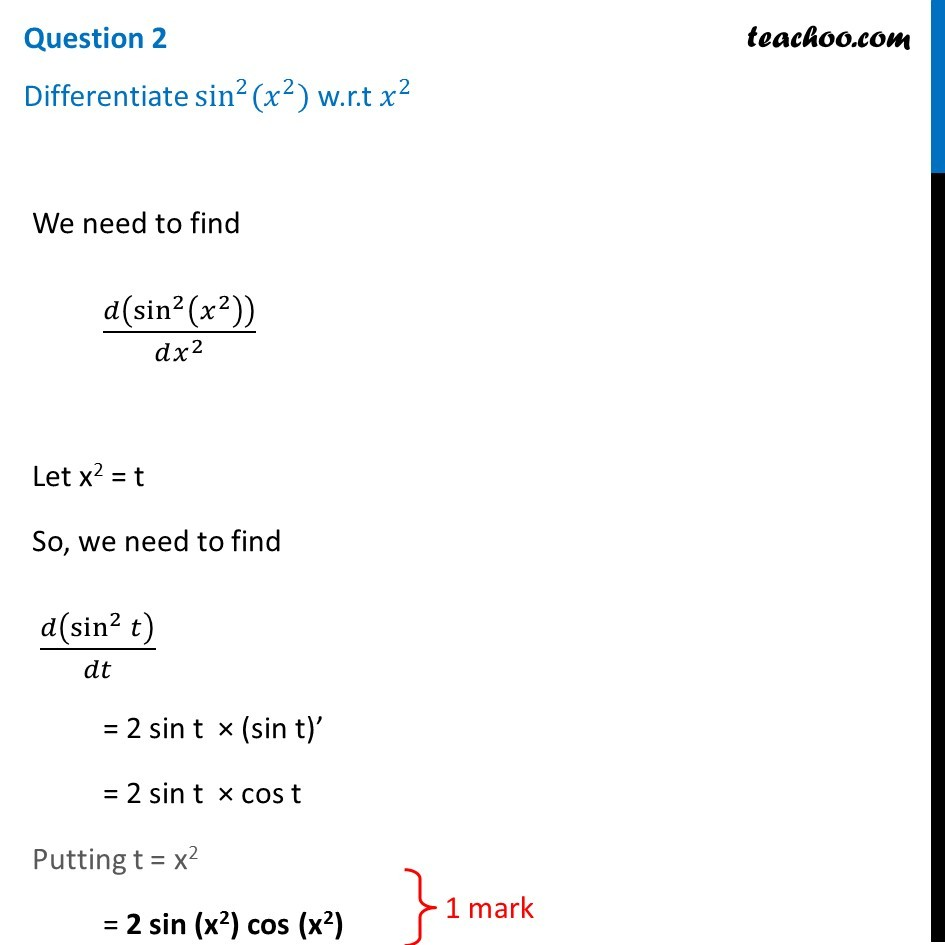Question 2

Differentiate sin 2 ⁡ (x 2 )  w.r.t x 21. Class 12
2. Solutions of Sample Papers and Past Year Papers - for Class 12 Boards
3. CBSE Class 12 Sample Paper for 2019 Boards

Transcript

Question 2 Differentiate sin^2⁡〖(𝑥^2)〗 w.r.t 𝑥^2 We need to find 𝑑(sin^2⁡(𝑥^2 ) )/(𝑑𝑥^2 ) Let x2 = t So, we need to find 𝑑(sin^2⁡𝑡 )/𝑑𝑡 = 2 sin t × (sin t)’ = 2 sin t × cos t Putting t = x2 = 2 sin (x2) cos (x2)

CBSE Class 12 Sample Paper for 2019 Boards

Class 12
Solutions of Sample Papers and Past Year Papers - for Class 12 Boards

About the AuthorDavneet Singh
Davneet Singh is a graduate from Indian Institute of Technology, Kanpur. He has been teaching from the past 10 years. He provides courses for Maths and Science at Teachoo.